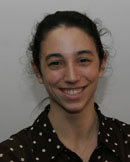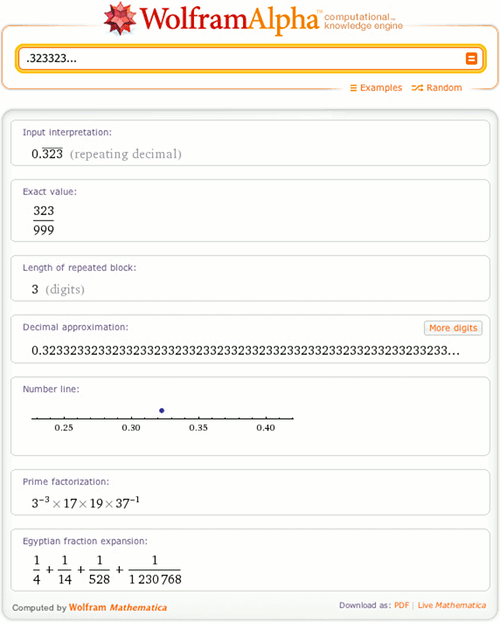# Repeating Decimals in Wolfram|Alpha

July 27, 2011 —Comments Off

In recent dinner conversation with my brother, I commented that I was “99.9 repeating” percent sure that my favorite author, Jorge Luis Borges, had lived into the 1980s (Wolfram|Alpha later showed me that he did, in fact, live through 1986!). I felt confident about my knowledge, but wanted to leave myself a little wiggle room, just in case. My brother grinned at me and said, “I know you know your geometric sequences. If you say you’re 99.9 repeating percent sure, then you’re 100 percent sure.”

I blushed, embarrassed. He was definitely right!

But just what did my brother mean? Well, he reminded me that:

99.999… = 99 + 9/10 + 9/100 + 9/1000 + 9/10000…

Glancing at the above, the series 9/10 + 9/100 + 9/1000 + 9/10000… stands out. Each term in this series is 1/10 times the term before it, making it a geometric series. A geometric series is a series wherein each term in the sequence is a constant number, r, multiplied by the term before it. Any geometric series whose r satisfies -1 < r < 1 is a convergent series, and we can say to what the series converges:

Sum[r^k, {k, 0, ∞}] = 1/(1-r)

Our sequence can be written as a geometric series as follows:

Sum[9/10 * (1/10)^k, {k, 0, ∞}]
= (9/10) * Sum[(1/10)^k, {k, 0, ∞}]

and we can use our geometric series formula:

(9/10) * Sum[1/10)^k, {k, 0, ∞}]
= (9/10) * 1/(1-1/10)
= (9/10) * (10/9)
= 1

So, here we have found that .999… = 1, meaning that 99.999… = 100 (and my brother was right). Next time, I will say that I am 99.9 percent sure instead!

The next natural step is to guess that the number 99.999… is not the only number of its kind. Every real number with a sequence of digits that repeats at some point after the decimal is called a “repeating decimal”. For example, the numbers .333… and .123501040104… are both repeating decimals. And because of their relationship to geometric sequences, every repeating decimal is equal to a rational number (and every rational number can be expressed as a repeating decimal). Let’s look at some other examples of repeating decimals in Wolfram|Alpha.One particularly cool repeating decimal is 1/7 = .142857…. Let’s look at this number a bit more:

Using Wolfram|Alpha, we see that when we multiply this number by any integer (other than a multiple of 7), the digits in the resulting decimal are a cyclic permutation of the number’s original digits. This means that 142857 is a cyclic number. More generally, for any prime, p, such that the length of the repeated block of 1/p is p-1, then the repeated block of 1/p is a cyclic number. Can you find any other cyclic numbers using repeating decimals in Wolfram|Alpha?

With its new support for repeating decimals, Wolfram|Alpha can help you with all of your repeating decimal questions, be they in the area of mathematical exploration or of casual conversation!

Very interesting post!

Posted by NDBilladeau July 27, 2011 at 4:41 pm

5882352941176470
-the sixteen digit cyclic number disclosed inside 1/17

Posted by Christel July 28, 2011 at 1:32 am

Humbug!

Posted by Lary July 29, 2011 at 9:58 pm

Here is another interesting analysis.

Case in point: Say you have: 99.9999999999….

— talking percents here —

Let A = 0.9999999999….

=> 100A = 99.9999999999….

=> 100A – A = 99

But 100A – A = 99A

=> So….99A = 99
=> A = 1

which then means 100*1 = 99.9999999999….

– or –

99.9999999999…. = 100

So…..99.9999999999….% IS 100%

Posted by fizixx August 6, 2011 at 2:14 pm

The fraction I was interested in today was 1/4. If I remember correctly, that was an old friends birthday.

Posted by Jeromy Mogharbel January 5, 2012 at 1:03 am

This surprised me at first. I thought perhaps I could use it to make some mathematical fallacy, but instead found another interesting connection. I started experimenting with repeating decimals.
1/9 = 0.11111…
2/9 = 0.22222…
3/9 = 0.33333… And so on.
Extending this pattern, what ratio should yield 0.99999… (the equivalent of 99 point 9 percent repeating)?
Answer: 9/9, which actually equals 1. I am sure I am not the first person to make this connection, but I thought it was neat.

Posted by Keith May 9, 2012 at 9:32 am

It’s late but this could be a more general proof:

Consider N = g1.g2g3…gkd1d2…djd1d2…dj… where gi and di are natural numbers

N*10^(k-1) = g1g2…gk.d1d2…dj…
= G + 0.A* where G = g1…gk and A* = d1…djd1…dj…

10^jA* = d1…dj.A*
(10^j-1)A* = d1…dj
A* = (d1…dj)/(10^j-1)

N*10^(k-1) = G + 10^-1(d1…dj)/(10^j-1)
N = 10^(-k)(G+M/(10^(j)-1)) where M = d1…dj

Posted by Shaon June 25, 2013 at 11:52 am

here’s one: 10,000 / 365

Posted by Les April 26, 2015 at 9:30 pm